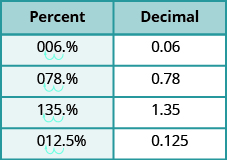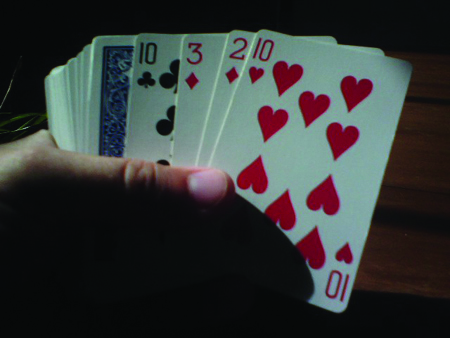## Writing Percents as Fractions and Decimals

### Learning Outcomes

• Convert a percent to a fraction
• Convert a percent to a decimal

## Percents —-> Fractions

Since percents are ratios, they can easily be expressed as fractions. Remember that percent means per $100$, so the denominator of the fraction is $100$.

### Convert a percent to a fraction

1. Write the percent as a ratio with the denominator $100$.
2. Simplify the fraction if possible.

### example

Convert each percent to a fraction:

1. $\text{36%}$
2. $\text{125%}$

Solution

 1. $36\%$ Write as a ratio with denominator $100$. ${\Large\frac{36}{100}}$ Simplify. ${\Large\frac{9}{25}}$
 2. $125\%$ Write as a ratio with denominator $100$. ${\Large\frac{125}{100}}$ Simplify. ${\Large\frac{5}{4}}$

### try it

The previous example shows that a percent can be greater than $1$. We saw that $\text{125%}$ means ${\Large\frac{125}{100}}$, or ${\Large\frac{5}{4}}$. These are improper fractions, and their values are greater than one.

### Example

Convert each percent to a fraction:

1. $\text{24.5%}$
2. $33\Large\frac{1}{3}\normalsize\%$

Solution

 1. $24.5\%$ Write as a ratio with denominator $100$. ${\Large\frac{24.5}{100}}$ Clear the decimal by multiplying numerator and denominator by $10$. ${\Large\frac{24.5\left(10\right)}{100\left(10\right)}}$ Multiply. ${\Large\frac{245}{1000}}$ Rewrite showing common factors. ${\Large\frac{5\cdot {49}}{5\cdot {200}}}$ Simplify. ${\Large\frac{49}{200}}$
 2. $33\Large\frac{1}{3}\normalsize\%$ Write as a ratio with denominator $100$. ${\Large\frac{33\Large\frac{1}{3}}{100}}$ Write the numerator as an improper fraction. ${\Large\frac{\frac{100}{3}}{100}}$ Rewrite as fraction division, replacing $100$ with $\frac{100}{1}$ ${\Large\frac{100}{3}}\div {\Large\frac{100}{1}}$ Multiply by the reciprocal. ${\Large\frac{100}{3}} \cdot {\Large\frac{1}{100}}$ Simplify. ${\Large\frac{1}{3}}$

## Percents —-> Decimals

In Decimals, we learned how to convert fractions to decimals. To convert a percent to a decimal, we first convert it to a fraction and then change the fraction to a decimal.

### Convert a percent to a decimal

1. Write the percent as a ratio with the denominator $100$.
2. Convert the fraction to a decimal by dividing the numerator by the denominator.

### example

Convert each percent to a decimal:

1. $\text{6%}$
2. $\text{78%}$

### try it

Convert each percent to a decimal:

1. $\text{9%}$
2. $\text{87%}$

### example

Convert each percent to a decimal:

1.  $\text{135%}$
2.  $\text{12.5%}$

Solution

 1. $135\%$ Write as a ratio with denominator $100$. ${\Large\frac{135}{100}}$ Change the fraction to a decimal by dividing the numerator by the denominator. $1.35$
 2. $12.5\%$ Write as a ratio with denominator $100$. ${\Large\frac{12.5}{100}}$ Change the fraction to a decimal by dividing the numerator by the denominator. $0.125$

### try it

Let’s summarize the results from the previous examples in the table below, and look for a pattern we could use to quickly convert a percent number to a decimal number.

Percent Decimal
$\text{6%}$ $0.06$
$\text{78%}$ $0.78$
$\text{135%}$ $1.35$
$\text{12.5%}$ $0.125$

Do you see the pattern?

To convert a percent number to a decimal number, we move the decimal point two places to the left and remove the $%$ sign. (Sometimes the decimal point does not appear in the percent number, but just like we can think of the integer $6$ as $6.0$, we can think of $\text{6%}$ as $\text{6.0%}$.) Notice that we may need to add zeros in front of the number when moving the decimal to the left.

The next table uses the percents in the table above and shows visually how to convert them to decimals by moving the decimal point two places to the left.### example

Among a group of business leaders, $\text{77%}$ believe that poor math and science education in the U.S. will lead to higher unemployment rates.

1. Convert the percent to a fraction
2. Convert the percent to a decimal

### Example

There are four suits of cards in a deck of cards—hearts, diamonds, clubs, and spades. The probability of randomly choosing a heart from a shuffled deck of cards is $\text{25%}$. Convert the percent to:

1. a fraction
2. a decimal

Solution

 1. $25\%$ Write as a ratio with denominator $100$. ${\Large\frac{25}{100}}$ Simplify. ${\Large\frac{1}{4}}$
 2. ${\Large\frac{1}{4}}$ Change the fraction to a decimal by dividing the numerator by the denominator. $0.25$(credit: Riles32807, Wikimedia Commons)

### try it

In summary, here is the table from above with conversions of percents to fractions added.

Percent Decimal Fraction
$\text{6%}$ $0.06$ ${\Large\frac{3}{50}}$
$\text{78%}$ $0.78$ ${\Large\frac{39}{50}}$
$\text{135%}$ $1.35$ ${\Large\frac{27}{20}}$
$\text{12.5%}$ $0.125$ ${\Large\frac{1}{8}}$